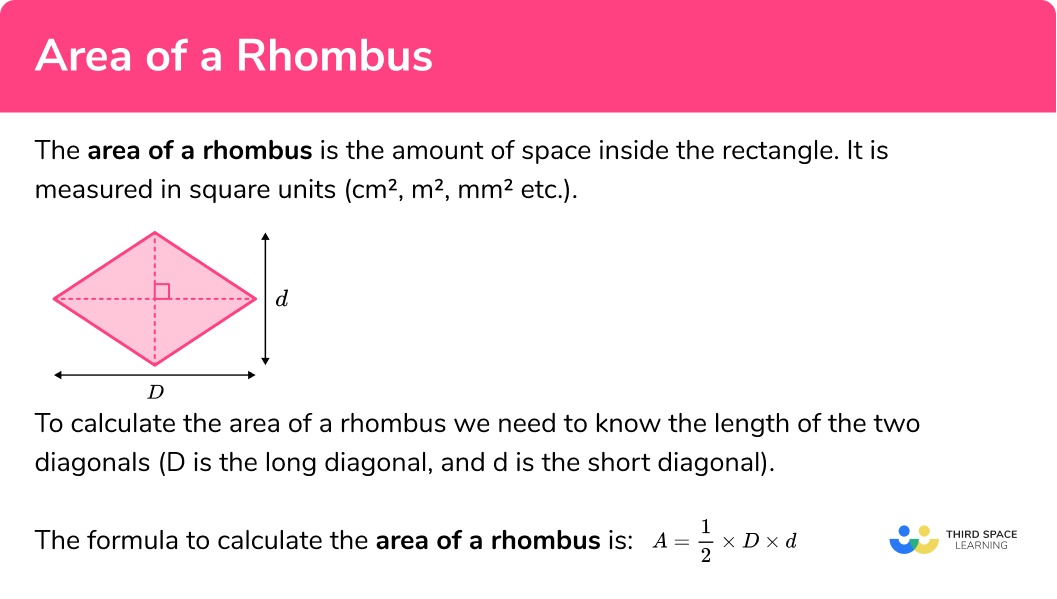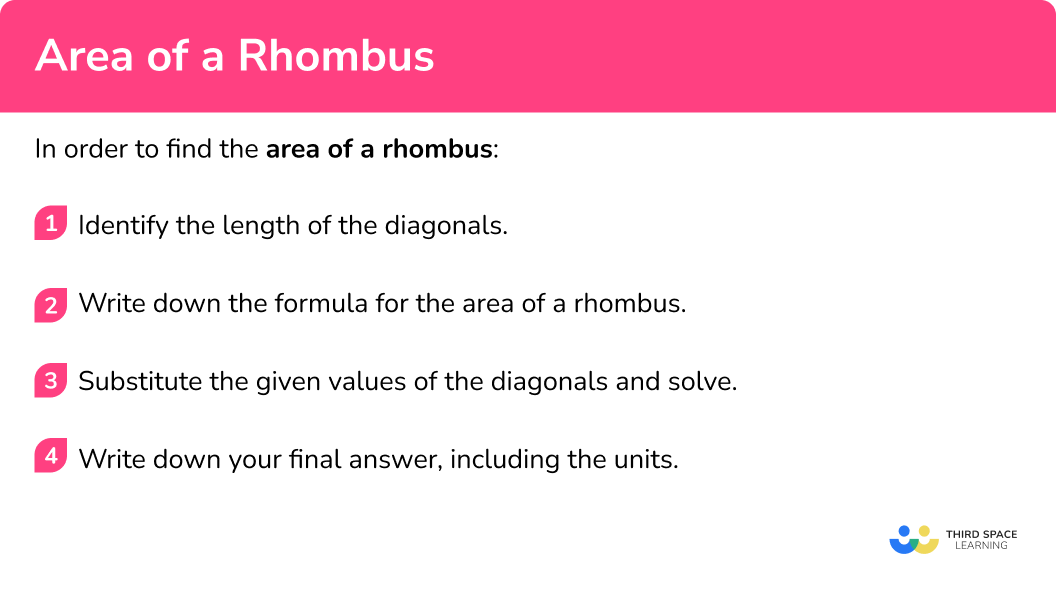# Area Of A Rhombus

Here we will learn about finding the area of a rhombus, including compound area questions, questions with missing side lengths and questions involving unit conversion.

There are also area of a rhombus worksheets based on Edexcel, AQA and OCR exam questions, along with further guidance on where to go next if you’re still stuck.

## What is the area of a rhombus?

The area of a rhombus is the amount of space inside the rhombus. It is measured in units squared ( cm^2, \ m^2, \ mm^2 etc).

Area formula:

A=\frac{1}{2}\times{D}\times{d}

Where D is the long diagonal of the rhombus (the base) and d is the short diagonal in the rhombus (the height of the rhombus).

A rhombus is a polygon and a quadrilateral ( 4 sided shape) where opposite sides are parallel, all sides are equal length and opposite angles are equal. The interior angles of a rhombus add up to 360^{\circ} . A rhombus is a special type of a parallelogram.

The diagonals of a parallelogram bisect each other at a right angle as shown below:

To calculate the area of a rhombus, we need to know the length of each diagonal of the rhombus.

If we move the two triangles from half of the rhombus to the opposing side, we get a rectangle:

The width of the rectangle is half of the long diagonal of the rhombus ( D ), and the height of the rectangle is the length of the short diagonal in the rhombus ( d ).

This gives the area of the rhombus formula:

A=\frac{1}{2}\times{D}\times{d}

### What is the area of a rhombus?## How to find the area of a rhombus

In order to find the area of a rhombus:

1. Identify the length of the diagonals.
2. Write down the formula for the area of a rhombus.
3. Substitute the given values of the diagonals and solve.

### How to find the area of a rhombus## Related lessons on area

Area of a rhombus is part of our series of lessons to support revision on area. You may find it helpful to start with the main area lesson for a summary of what to expect, or use the step by step guides below for further detail on individual topics. Other lessons in this series include:

## Area of a rhombus examples

### Example 1: finding area given the length of the diagonals

Find the area of the rhombus below:

1. Identify the length of the diagonals.

\begin{aligned} &AC = 2m \\\\ &BD = 9m \end{aligned}

These are the diagonals of a rhombus which correspond to the base and height of the corresponding rectangle.

2Write down the formula for the area of a rhombus.

A=\frac{1}{2}\times{D}\times{d}

3Substitute the given values of the diagonals and solve.

\begin{aligned} A&=\frac{1}{2}\times{D}\times{d}\\\\ &=\frac{1}{2}\times{9}\times{2}\\\\ &=9 \end{aligned}

In this case we are working with metres so our final answer must be in square metres.

A=9m^2

### Example 2: finding the area of a rhombus requiring converting units

Calculate the area of the rhombus below:

Identify the length of the diagonals.

Write down the formula for the area of a rhombus.

Substitute the given values of the diagonals and solve.

### Example 3: worded problem

A rhombus ABCD has the point O as the point of intersection of the two diagonals AC and BD . The length OB=3cm , and OC=5cm . Calculate the area of the rhombus.

Identify the length of the diagonals.

Write down the formula for the area of a rhombus.

Substitute the given values of the diagonals and solve.

### Example 4: calculating the diagonal given area and the other diagonal

Calculate the length of AC given the length of BD=14m and the area of the rhombus is 35m^2 . Use the diagram below to help you.

Identify the length of the diagonals.

Write down the formula for the area of a rhombus.

Substitute the given values of the diagonals and solve.

### Example 5: area given side length and one diagonal

ABCD is a rhombus where the edges AB and CD are parallel. Given that AB=20cm and BD=32cm, calculate the area of the rhombus.

Identify the length of the diagonals.

Write down the formula for the area of a rhombus.

Substitute the given values of the diagonals and solve.

### Example 6: calculate the area using trigonometry (higher only)

A rhombus has a side length of 13cm . One pair of interior angles is double the other pair of interior angles. Calculate the area of the rhombus to 2 decimal places.

Identify the length of the diagonals.

Write down the formula for the area of a rhombus.

Substitute the given values of the diagonals and solve.

### Common misconceptions

• Using incorrect units for the answer

A common error is to forget to include squared units when asked to calculate area.

• Forgetting to convert measures to a common unit

Before using the formula for calculating the area of a rectangle we need to ensure that units are the same. If different units are given (E.g. length = 4m and width = 3cm ) then you must convert them either both to cm or both to m.

• Using half of the diagonal

As the area of a rhombus is half the length of one diagonal multiplied by the length of the other diagonal, the other diagonal is also halved within the calculation, leaving a solution that is out by a factor of 2.

• Calculating the area of a square (using side lengths)

The area of a rhombus is calculated by using the side lengths, instead of the lengths of the diagonals.

### Practice area of a rhombus questions

1. Find the area of the rhombus below:24m^248m^216m^248m\begin{aligned} A&=\frac{1}{2}\times{D}\times{d}\\\\ &=\frac{1}{2}\times{12}\times{4}\\\\ &=24 \end{aligned}

A=24\text{ m}^2

2. The rhombus MNOP has the following information:

MP=250cm

NO=4.5m

Calculate the area of the rhombus in square centimetres.

11250cm^228125cm^256.25cm^256250cm^2\begin{aligned} A&=\frac{1}{2}\times{D}\times{d}\\\\ &=\frac{1}{2}\times{250}\times{450}\\\\ &=56250 \end{aligned}

A=56250\text{ cm}^2

3. Mr. Perry owns a farm and is looking to make the chicken enclosure shown below. Each chicken needs 4 square metres to move around freely. What is the maximum number of chickens that can fit into the enclosure?244896192Calculating the area of the rhombus, we have

\begin{aligned} A&=\frac{1}{2}\times{D}\times{d}\\\\ &=\frac{1}{2}\times{12}\times{16}\\\\ &=96 \end{aligned}

A=96\text{ m}^2

As the field is 96m^2 and each chicken requires 4m^2, \ 96\div{4}=24 chickens

4. The area of rhombus ABCD is 54m^2. The centre of the rhombus is located at point P . Given that AP=3m , calculate the length BD.9m18m54m324mAs A=54m^2 and AP=3m, \ AC=32=6m.

Using the formula for the area of a rhombus, we have

\begin{aligned} A&=\frac{1}{2}\times{D}\times{d}\\\\ 54&=\frac{1}{2}\times{6}\times{x}\\\\ 54&=3x\\\\ x&=18 \end{aligned}

x=18\text{ m}

5. In the rhombus below AB = 5m, \ BD = 8m. Calculate the area of the rhombus.24m^248m^26m^220m^2We can calculate half the length of AC by using Pythagoras’ theorem. Let M be the centre of the rhombus. The distance BM is half the distance BD and so BM=4m.Using Pythagoras’ theorem to calculate the length of AM , we have:

\begin{aligned} AM^{2}&=5^{2}-4^{2}\\\\ &=25-16\\\\ &=9\\\\ AM&=3 \end{aligned}

As AM=3m, \ AC=3 \times 2=6m.

The area of the rhombus is therefore:

\begin{aligned} A&=\frac{1}{2}\times{D}\times{d}\\\\ &=\frac{1}{2}\times{6}\times{8}\\\\ &=24 \end{aligned}

A=24\text{ m}^2

6. (Higher only) A rhombus PQRS has side length 5cm . The rhombus has two pairs of interior angles, equal to x and 3x-20 .

Use the cosine rule c^2=a^2+b^2-2ab \cos(\theta) to calculate the area of the rhombus to 1 decimal place.

19.2cm^212.5cm^225.0cm^220.0cm^2First we need to calculate the value of the two pairs of angles in the rhombus:

\begin{aligned} x+3x-20+x+3x-20&=360\\\\ 8x-40&=360\\\\ 8x&=400\\\\ x&=50 \end{aligned}

x=50^{\circ}

3x-20=130^{\circ}

So we have a rhombus with a side length of 5cm and the interior angles are 50^{\circ} and 130^{\circ}.Calculating the distance PR using the cosine rule, we have:

PR=\sqrt{5^{2}+5^{2}-2\times{5}\times{5}\times\cos(130)}=9.06307787

Calculating the distance QS using the cosine rule, we have:

QS=\sqrt{5^{2}+5^{2}-2\times{5}\times{5}\times\cos(50)}=4.226182617

The area of the rhombus is therefore:
\begin{aligned} A&=\frac{1}{2}\times{D}\times{d}\\\\ &=\frac{1}{2}\times{9.06307787}\times{4.226182617}\\\\ &=19.15111108 \end{aligned}

A=19.2\text{ cm}^2\text{ (1dp)}

### Area of a rhombus GCSE questions

1. Shown below is a rhombus where AM=6m , and BM=10m . Calculate the area of the rhombus.(3 marks)

AC=12m, \ BD=20m

(1)

\frac{12 \times 20}{2}

(1)

120m^2

(1)

2. (a) A rectangular logo is composed of 8 congruent rhombuses. Calculate the area of one rhombus.(b) Calculate the perimeter of the logo. Write your answer as a surd in its simplest form.

(6 marks)

(a)

12\div{3}=4\text{m and }4\div{2}=2\text{m}

(1)

\frac{4 \times 2}{2}

(1)

4\text{ m}^{2}

(1)

(b)

2^{2}+1^{2}=5

(1)

Side length of a rhombus =\sqrt{5}

(1)

16\sqrt{5}

(1)

3. Ms. Polly is looking to cover her garden with new grass. One roll of grass covers 6 square metres and costs £9. How much would it cost to cover the entire ground with grass?(5 marks)

\sqrt{25^{2}-7^{2}}

(1)

24m

(1)

\frac{48 \times 14}{2}

(1)

(336 \div 6) \times 9

(1)

£504

(1)

## Learning checklist

You have now learned how to:

• Calculate the area of a rhombus and related composite shapes
• Calculate the area of parallelograms
• Recognise when it is possible to use formulae for area
• Derive and apply formulae to calculate and solve problems involving: perimeter and area of triangles and parallelograms

## Still stuck?

Prepare your KS4 students for maths GCSEs success with Third Space Learning. Weekly online one to one GCSE maths revision lessons delivered by expert maths tutors.

Find out more about our GCSE maths tuition programme.